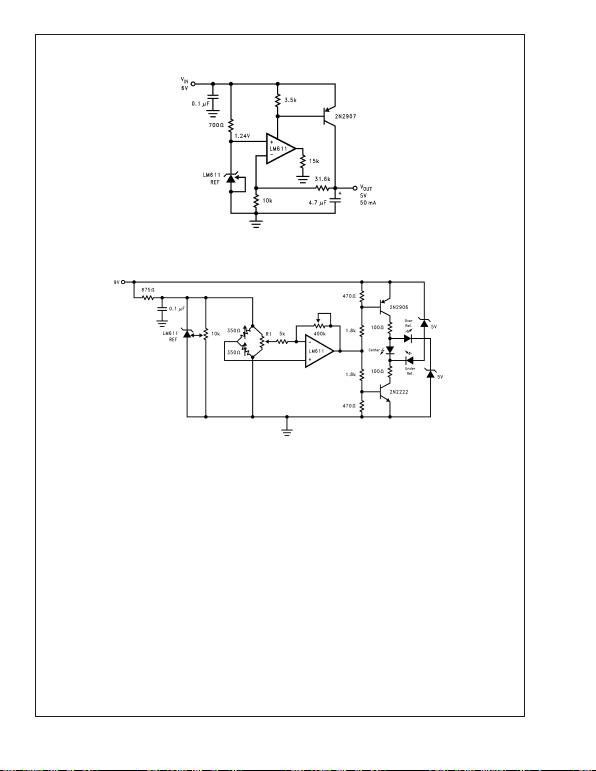LM611
LM611 Operational Amplifier and Adjustable Reference
Literature Number: SNOSC08B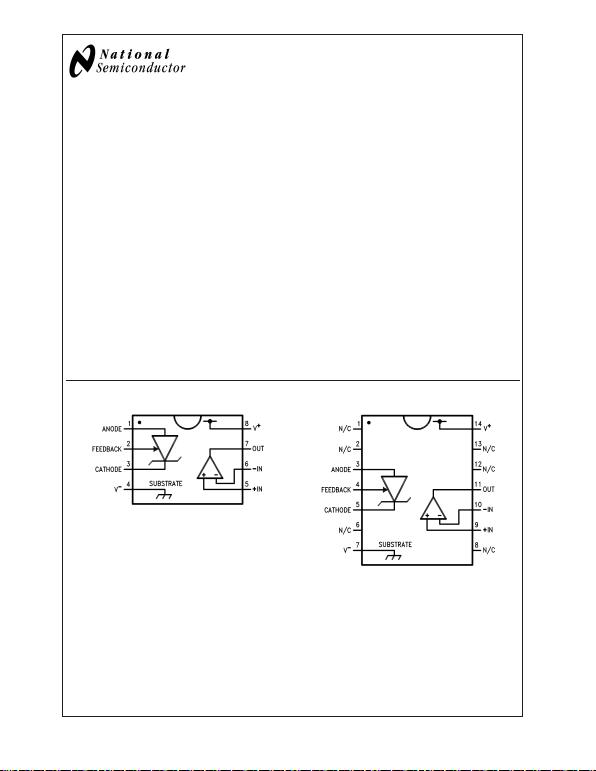LM611
General Description
The LM611 consists of a single-supply op-amp and a pro-
grammable voltage reference in one space saving 8-pin
package. The op-amp out-performs most single-supply op-
amps by providing higher speed and bandwidth along with
low supply current. This device was specifically designed to
lower cost and board space requirements in transducer, test,
measurement and data acquisition systems.
Combining a stable voltage reference with a wide output
swing op-amp makes the LM611 ideal for single supply
transducers, signal conditioning and bridge driving where
large common-mode signals are common. The voltage ref-
erence consists of a reliable band-gap design that maintains
low dynamic output impedance (1typical), excellent initial
tolerance (0.6%), and the ability to be programmed from
1.2V to 6.3V via two external resistors. The voltage refer-
ence is very stable even when driving large capacitive loads,
as are commonly encountered in CMOS data acquisition
systems.
As a member of National’s Super-Blockfamily, the LM611
is a space-saving monolithic alternative to a multi-chip solu-
tion, offering a high level of integration without sacrificing
performance.
Features
OP AMP
nLow operating current: 300 µA (op amp)
nWide supply voltage range: 4V to 36V
nWide common-mode range: V
to (V
+
−1.8V)
nWide differential input voltage: ±36V
nAvailable in low cost 8-pin DIP
nAvailable in plastic package rated for Military
Temperature Range Operation
REFERENCE
nAdjustable output voltage: 1.2V to 6.3V
nTight initial tolerance available: ±0.6%
nWide operating current range: 17 µA to 20 mA
nReference floats above ground
Applications
nTransducer bridge driver
nProcess and Mass Flow Control systems
nPower supply voltage monitor
nBuffered voltage references for A/D’s
Connection Diagrams
00922101
00922102
Super-Blockis a trademark of National Semiconductor Corporation.
August 2000
LM611 Operational Amplifier and Adjustable Reference
© 2004 National Semiconductor Corporation DS009221 www.national.com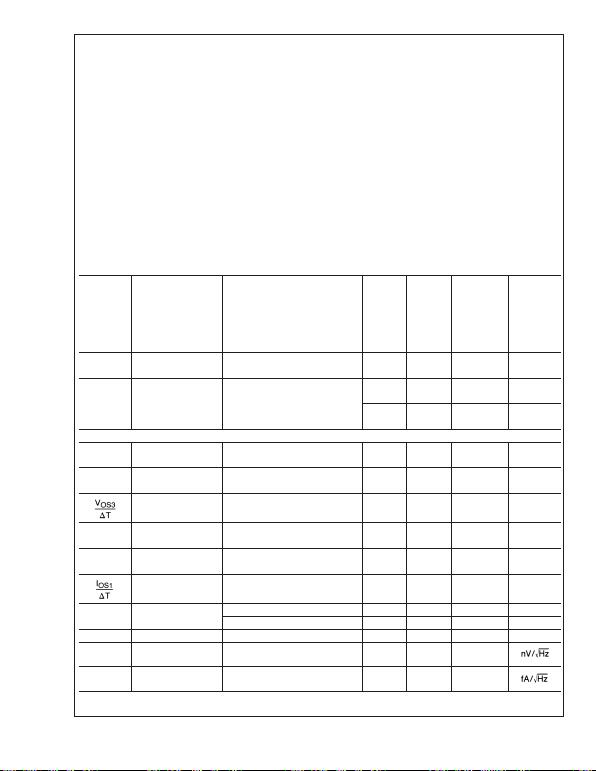Absolute Maximum Ratings (Note 1)
If Military/Aerospace specified devices are required,
Distributors for availability and specifications.
Voltage on Any Pins Except V
R
(referred to V
pin) 36V (Max)
(Note 2) −0.3V (Min)
Current through Any Input Pin
and
V
R
Pin ±20 mA
Differential Input Voltage
Military and Industrial ±36V
Commercial ±32V
Storage Temperature Range −65˚CT
J
+150˚C
Maximum Junction Temperature 150˚C
Thermal Resistance, Junction-to-Ambient (Note 3)
N Package 100˚C/W
M Package 150˚C/W
Soldering Information Soldering (10 seconds)
N Package 260˚C
M Package 220˚C
ESD Tolerance (Note 4) ±1kV
Operating Temperature Range
LM611AI, LM611I, LM611BI −40˚CT
J
+85˚C
LM611AM, LM611M −55˚CT
J
+125˚C
LM611C 0˚CT
J
70˚C
Electrical Characteristics
These specifications apply for V
= GND = 0V, V
+
= 5V, V
CM
=V
OUT
= 2.5V, I
R
= 100 µA, FEEDBACK pin shorted to GND,
unless otherwise specified. Limits in standard typeface are for T
J
= 25˚C; limits in boldface type apply over the Operating
Temperature Range.
LM611M
LM611AM LM611BI
Symbol Parameter Conditions Typical LM611AI LM611I Units
(Note 5) Limits LM611C
(Note 6) Limits
(Note 6)
I
S
Total Supply Current R
=, 210 300 350 µA max
4V V
+
36V (32V for LM611C) 221 320 370 µA max
V
S
Supply Voltage Range 2.2 2.8 2.8 V min
2.9 3 3 V min
46 36 32 V max
43 36 32 V max
OPERATIONAL AMPLIFIER
V
OS1
V
OS
Over Supply 4V V
+
36V 1.5 3.5 5.0 mV max
(4V V
+
32V for LM611C) 2.0 6.0 7.0 mV max
V
OS2
V
OS
Over V
CM
V
CM
= 0V through V
CM
= 1.0 3.5 5.0 mV max
(V
+
1.8V), V
+
= 30V, V
=0V 1.5 6.0 7.0 mV max
Average V
OS
Drift (Note 6) 15 µV/˚C
max
I
B
Input Bias Current 10 25 35 nA max
11 30 40 nA max
I
OS
Input Offset Current 0.2 4 4 nA max
0.3 5 5 nA max
Average Offset Drift
Current 4pA/˚C
R
IN
Input Resistance Differential 1800 M
Common-Mode 3800 M
C
IN
Input Capacitance Common-Mode 5.7 pF
e
n
Voltage Noise f = 100 Hz,
Input Referred
74
I
n
Current Noise f = 100 Hz,
Input Referred
58
LM611
www.national.com 2Electrical Characteristics (Continued)
These specifications apply for V
= GND = 0V, V
+
= 5V, V
CM
=V
OUT
= 2.5V, I
R
= 100 µA, FEEDBACK pin shorted to GND,
unless otherwise specified. Limits in standard typeface are for T
J
= 25˚C; limits in boldface type apply over the Operating
Temperature Range.
LM611M
LM611AM LM611BI
Symbol Parameter Conditions Typical LM611AI LM611I Units
(Note 5) Limits LM611C
(Note 6) Limits
(Note 6)
OPERATIONAL AMPLIFIER
CMRR Common-Mode V
+
= 30V, 0V V
CM
(V
+
1.8V) 95 80 75 dB min
Rejection-Ratio CMRR = 20 log (V
CM
/V
OS
)90 75 70 dB min
PSRR Power Supply 4V V
+
30V, V
CM
=V
+
/2, 110 80 75 dB min
Rejection-Ratio PSRR = 20 log (V
+
/V
OS
)100 75 70 dB min
A
V
Open Loop R
L
=10kto GND, V
+
= 30V, 500 100 94 V/mV
Voltage Gain 5V V
OUT
25V 50 40 40 min
SR Slew Rate V
+
= 30V (Note 7) 0.70 0.55 0.50 V/µs
0.65 0.45 0.45
GBW Gain Bandwidth C
L
= 50 pF 0.80 MHz
0.50
V
O1
Output Voltage R
L
=10kto GND V
+
1.4 V
+
1.7 V
+
1.8 V min
Swing High V
+
= 36V (32V for LM611C) V
+
1.6 V
+
1.9 V
+
1.9 V min
V
O2
Output Voltage R
L
=10kto V
+
V
+ 0.8 V
+ 0.9 V
+ 0.95 V max
Swing Low V
+
= 36V (32V for LM611C) V
+ 0.9 V
+ 1.0 V
+ 1.0 V max
I
OUT
Output Source V
OUT
= 2.5V, V
+IN
= 0V, 25 20 16 mA min
Current V
−IN
= −0.3V 15 13 13 mA min
I
SINK
Output Sink V
OUT
= 1.6V, V
+IN
= 0V, 17 14 13 mA min
Current V
−IN
= 0.3V 98 8mA min
I
SHORT
Short Circuit Current V
OUT
= 0V, V
+IN
= 3V, 30 50 50 mA max
V
−IN
= 2V, Source 40 60 60 mA max
V
OUT
= 5V, V
+IN
= 2V, 30 60 70 mA max
V
−IN
= 3V, Sink 32 80 90 mA max
VOLTAGE REFERENCE
V
R
Reference Voltage (Note 8) 1.244 1.2365 1.2191 V min
1.2515 1.2689 V max
(±0.6%) (±2.0%)
Average Temperature
Drift
(Note 9)
10 80 150
PPM/˚C
max
Hysteresis Hyst = (Vro' Vro)/T
J
(Note 10)
3.2 µV/˚C
V
R
Change V
R(100 µA)
−V
R(17 µA)
0.05 1 1 mV max
with Current 0.1 1.1 1.1 mV max
V
R(10 mA)
−V
R(100 µA)
1.5 5 5 mV max
(Note 11) 2.0 5.5 5.5 mV max
R Resistance V
R(100.1 mA)
/9.9 mA 0.2 0.56 0.56 max
V
R(10017 µA)
/83 µA 0.6 13 13 max
V
R
Change with V
R(Vro = Vr)
−V
R(Vro = 6.3V)
2.5 7 7 mV max
High V
RO
(5.06V between Anode and
FEEDBACK)
2.8 10 10 mV max
LM611
www.national.com3Electrical Characteristics (Continued)
These specifications apply for V
= GND = 0V, V
+
= 5V, V
CM
=V
OUT
= 2.5V, I
R
= 100 µA, FEEDBACK pin shorted to GND,
unless otherwise specified. Limits in standard typeface are for T
J
= 25˚C; limits in boldface type apply over the Operating
Temperature Range.
LM611M
LM611AM LM611BI
Symbol Parameter Conditions Typical LM611AI LM611I Units
(Note 5) Limits LM611C
(Note 6) Limits
(Note 6)
VOLTAGE REFERENCE
V
R
Change with V
R(V+ = 5V)
−V
R(V+ = 36V)
0.1 1.2 1.2 mV max
V
+
Change (V
+
= 32V for LM611C) 0.1 1.3 1.3 mV max
V
R(V+ = 5V)
−V
R(V+ = 3V)
0.01 1 1 mV max
0.01 1.5 1.5 mV max
V
R
Change with V
+
=V
+
max, V
R
=V
R
V
ANODE
Change (@V
ANODE
=V
=GND)−V
R
0.7 1.5 1.6 mV max
(@V
ANODE
=V
+
1.0V) 3.3 3.0 3.0 mV max
I
FB
FEEDBACK Bias I
FB
;V
ANODE
V
FB
5.06V 22 35 50 nA max
Current 29 40 55 nA max
e
n
V
R
Noise 10 Hz to 10,000 Hz, V
RO
=V
R
30 µV
RMS
Note 1: Absolute maximum ratings indicate limits beyond which damage to the component may occur. Electrical specifications do not apply when operating the
device beyond its rated operating conditions.
Note 2: More accurately, it is excessive current flow, with resulting excess heating, that limits the voltages on all pins. When any pin is pulled a diode drop below
V, a parasitic NPN transistor turns ON. No latch-up will occur as long as the current through that pin remains below the Maximum Rating. Operation is undefined
and unpredictable when any parasitic diode or transistor is conducting.
Note 3: Junction temperature may be calculated using TJ=T
A+P
DθJA. The given thermal resistance is worst-case for packages in sockets in still air. For packages
soldered to copper-clad board with dissipation from one op amp or reference output transistor, nominal θJA is 90˚C/W for the N package and 135˚C/W for the M
package.
Note 4: Human body model, 100 pF discharged through a 1.5 kresistor.
Note 5: Typical values in standard typeface are for TJ= 25˚C; values in boldface type apply for the full operating temperature range. These values represent the
most likely parametric norm.
Note 6: All limits are guaranteed at room temperature (standard type face) or at operating temperature extremes (bold face type).
Note 7: Slew rate is measured with op amp in a voltage follower configuration. For rising slew rate, the input voltage is driven from 5V to 25V, and the output voltage
transition is sampled at 10V and 20V. For falling slew rate, the input voltage is driven from 25V to 5V, and output voltage transition is sampled at 20V and 10V.
Note 8: VRis the cathode-feedback voltage, nominally 1.244V.
Note 9: Average reference drift is calculated from the measurement of the reference voltage at 25˚C and at the temperature extremes. The drift, in ppm/˚C, is
106VR/(VR[25˚C]TJ), where VRis the lowest value subtracted from the highest, VR[25˚C] is the value at 25˚C, and TJis the temperature range. This parameter
is guaranteed by design and sample testing.
Note 10: Hysteresis is the change in VRcaused by a change in TJ, after the reference has been “dehysterized”. To dehysterize the reference; that is minimize the
hysteresis to the typical value, its junction temperature should be cycled in the following pattern, spiraling in toward 25˚C: 25˚C, 85˚C, −40˚C, 70˚C, 0˚C, 25˚C.
Note 11: Low contact resistance is required for accurate measurement.
Note 12: Military RETS 611AMX electrical test specification is available on request. The LM611AMJ/883 can also be procured as a Standard Military Drawing.
LM611
www.national.com 4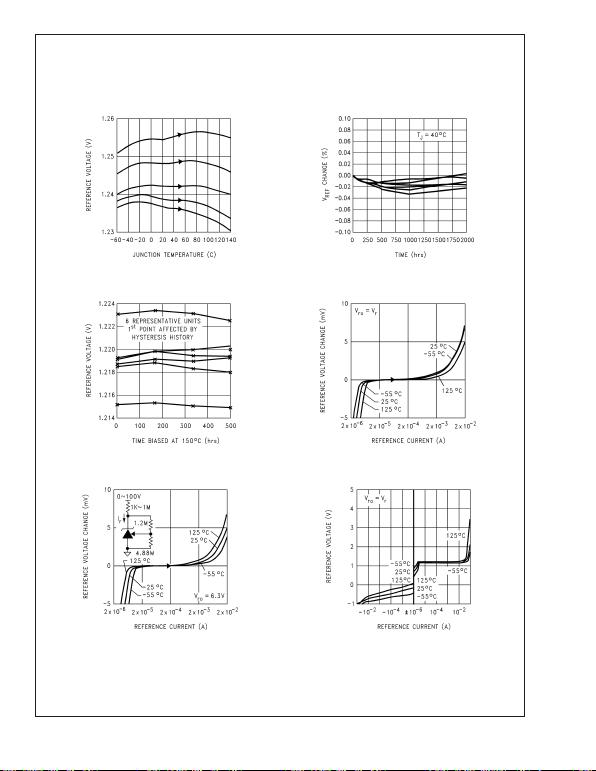Typical Performance Characteristics (Reference)
T
J
= 25˚C, FEEDBACK pin shorted to V
= 0V, unless
otherwise noted
Reference Voltage vs Temp
on 5 Representative Units Reference Voltage Drift
00922133 00922134
Accelerated Reference
Voltage Drift vs Time
Reference Voltage vs
Current and Temperature
00922135 00922136
Reference Voltage vs
Current and Temperature
Reference Voltage vs
Reference Current
00922137 00922138
LM611
www.national.com5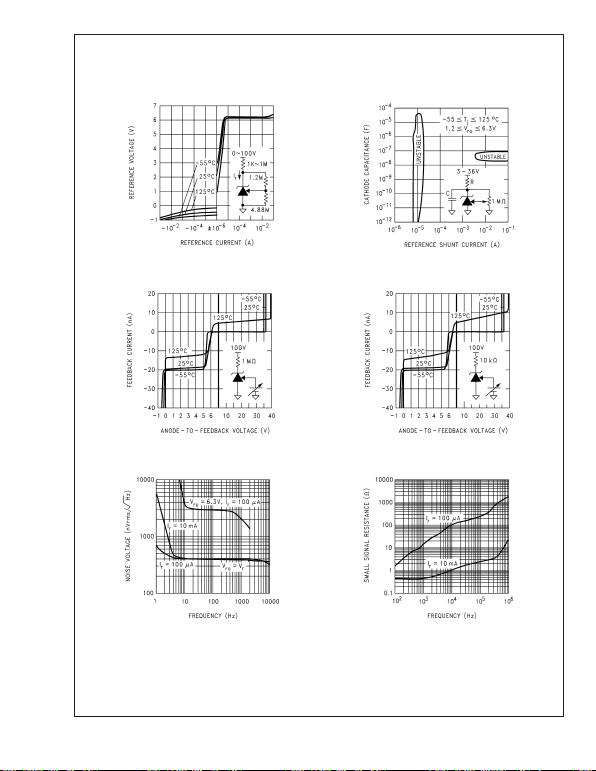Typical Performance Characteristics (Reference) T
J
= 25˚C, FEEDBACK pin shorted to V
=
0V, unless otherwise noted (Continued)
Reference Voltage vs
Reference Current
Reference AC
Stability Range
00922139 00922140
Feedback Current vs
Feedback-to-Anode Voltage
Feedback Current vs
Feedback-to-Anode Voltage
00922141 00922142
Reference Noise Voltage
vs Frequency
Reference Small-Signal
Resistance vs Frequency
00922143 00922144
LM611
www.national.com 6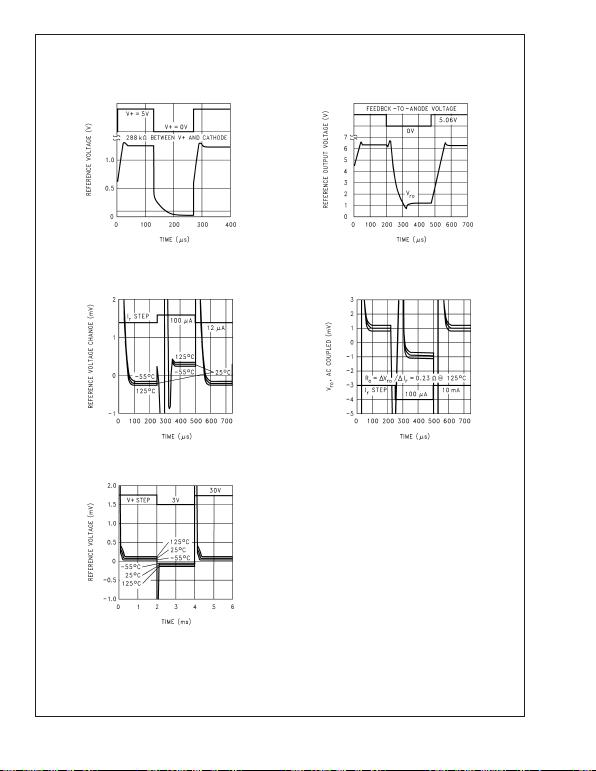Typical Performance Characteristics (Reference) T
J
= 25˚C, FEEDBACK pin shorted to V
=
0V, unless otherwise noted (Continued)
Reference Power-Up Time
Reference Voltage with
Feedback Voltage Step
00922145 00922146
Reference Voltage with
10012 µA Current Step
Reference Step Response
for 100 µA 10 mA
Current Step
00922147 00922148
Reference Voltage Change
with Supply Voltage Step
00922149
LM611
www.national.com7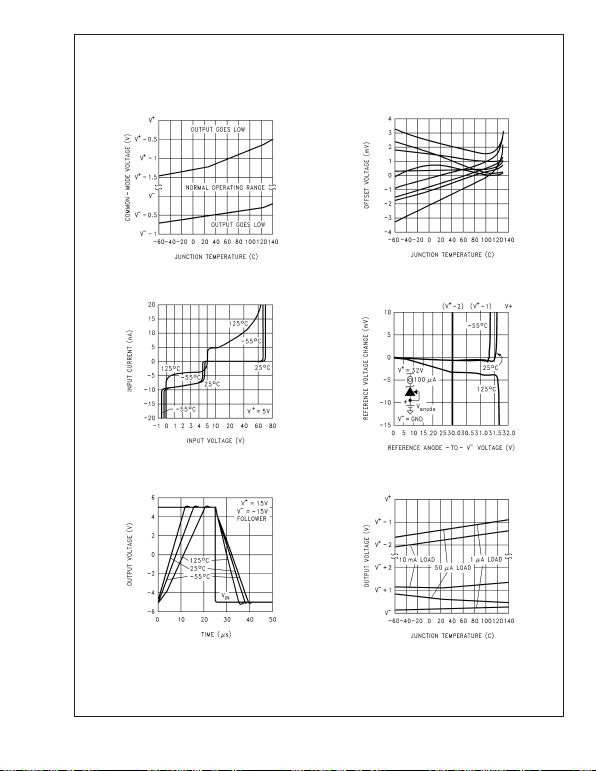Typical Performance Characteristics (Op Amps)
V
+
= 5V, V
= GND = 0V, V
CM
=V
+
/2, V
OUT
=V
+
/2, T
J
=
25˚C, unless otherwise noted
Input Common-Mode Voltage
Range vs Temperature
V
OS
vs Junction
Temperature
00922150 00922151
Input Bias Current vs
Common-Mode Voltage
Reference Change vs
Common-Mode Voltage
00922152
00922153
Large-Signal
Step Response
Output Voltage Swing
vs Temp. and Current
00922154 00922155
LM611
www.national.com 8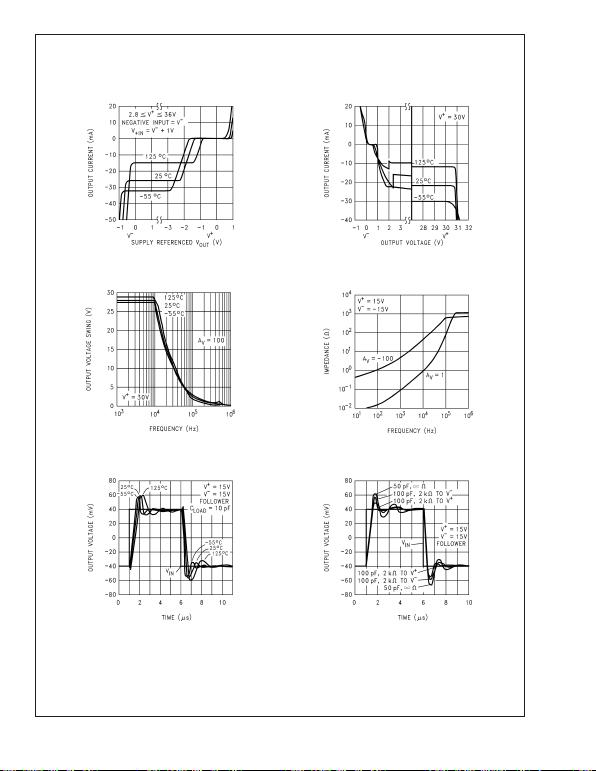Typical Performance Characteristics (Op Amps) V
+
= 5V, V
= GND = 0V, V
CM
=V
+
/2, V
OUT
=V
+
/2, T
J
= 25˚C, unless otherwise noted (Continued)
Output Source Current vs
Output Voltage and Temp.
Output Sink Current vs
Output Voltage
00922156 00922157
Output Swing,
Large Signal
Output Impedance vs
Frequency and Gain
00922158 00922159
Small Signal Pulse
Response vs Temp.
Small-Signal Pulse
00922160 00922161
LM611
www.national.com9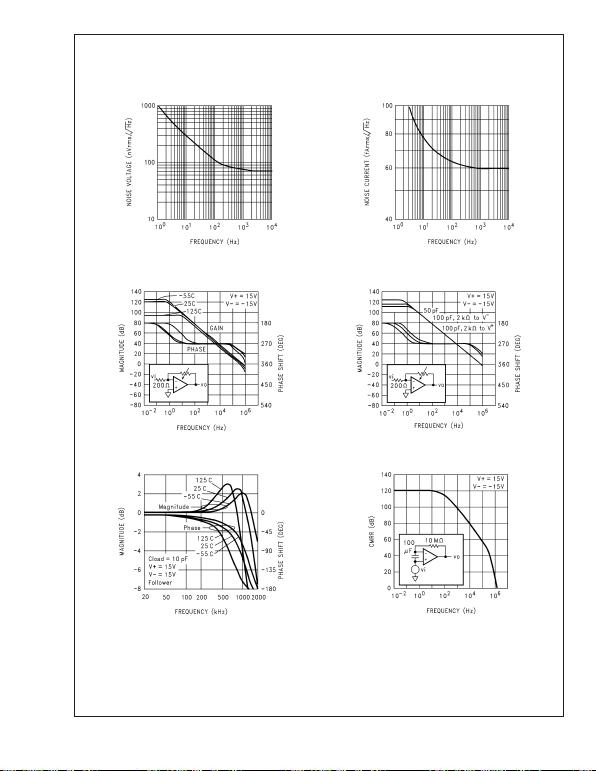Typical Performance Characteristics (Op Amps) V
+
= 5V, V
= GND = 0V, V
CM
=V
+
/2, V
OUT
=V
+
/2, T
J
= 25˚C, unless otherwise noted (Continued)
Op Amp Voltage Noise
vs Frequency
Op Amp Current Noise
vs Frequency
00922162 00922163
Small-Signal Voltage Gain vs
Frequency and Temperature
Small-Signal Voltage Gain
00922164 00922165
Follower Small-Signal
Frequency Response
Common-Mode Input
Voltage Rejection Ratio
00922166 00922167
LM611
www.national.com 10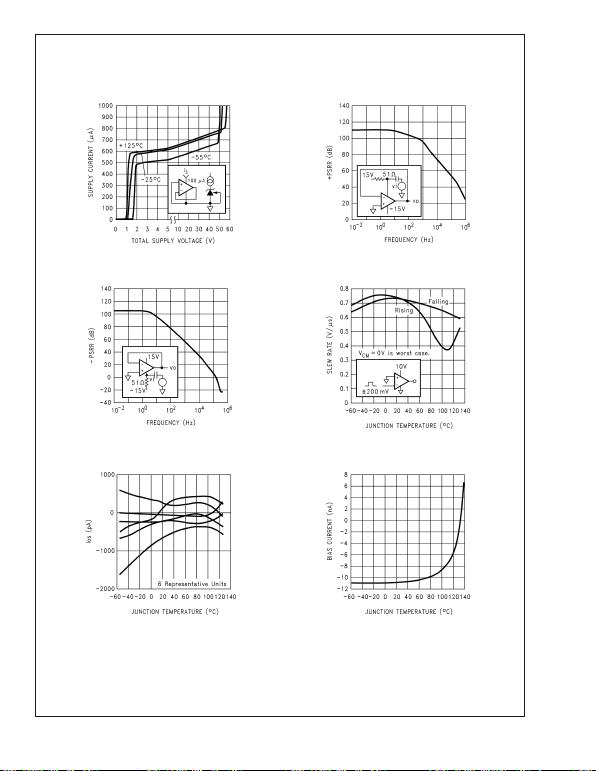Typical Performance Characteristics (Op Amps) V
+
= 5V, V
= GND = 0V, V
CM
=V
+
/2, V
OUT
=V
+
/2, T
J
= 25˚C, unless otherwise noted (Continued)
Power Supply Current vs
Power Supply Voltage
Positive Power Supply
Voltage Rejection Ratio
00922168 00922169
Negative Power Supply
Voltage Rejection Ratio Slew Rate vs Temperature
00922170 00922171
Input Offset Current vs
Junction Temperature
Input Bias Current vs
Junction Temperature
00922172 00922173
LM611
www.national.com11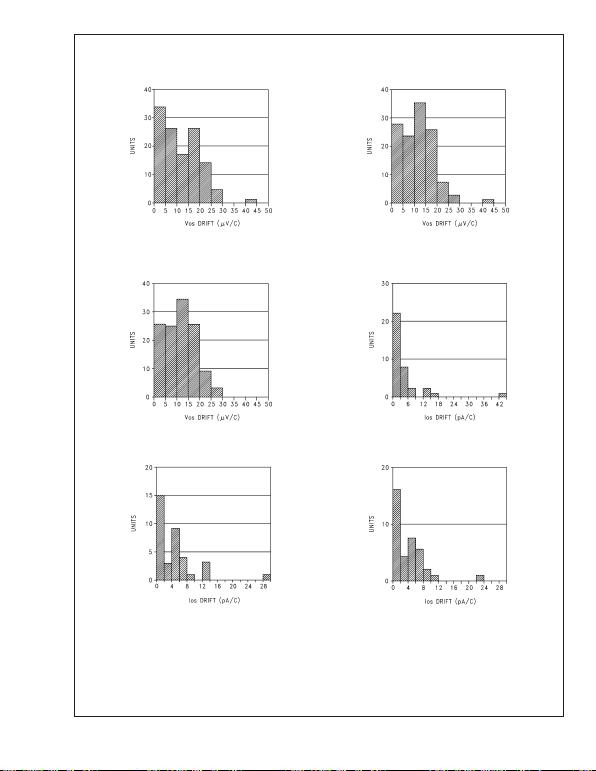Typical Performance Distributions
Average V
OS
Drift
Military Temperature Range
Average V
OS
Drift
Industrial Temperature Range
00922174 00922175
Average V
OS
Drift
Commercial Temperature
Range
Average I
OS
Drift
Military Temperature Range
00922176 00922177
Average I
OS
Drift
Industrial Temperature Range
Average I
OS
Drift
Commercial Temperature Range
00922178 00922179
LM611
www.national.com 12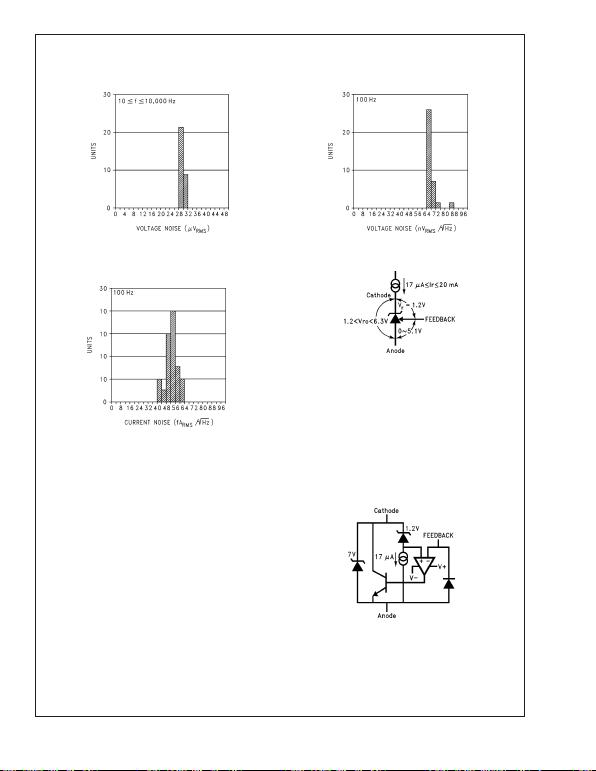Typical Performance Distributions (Continued)
Noise Distribution
Op Amp Voltage
Noise Distribution
00922180 00922181
Op Amp Current
Noise Distribution
00922182
Application Information
VOLTAGE REFERENCE
Reference Biasing
The voltage reference is of a shunt regulator topology that
models as a simple zener diode. With current I
r
flowing in the
‘forward’ direction there is the familiar diode transfer func-
tion. I
r
flowing in the reverse direction forces the reference
voltage to be developed from cathode to anode. The applied
voltage to the cathode may range from a diode drop below
V
to the reference voltage or to the avalanche voltage of the
parallel protection diode, nominally 7V. A 6.3V reference with
V+ = 3V is allowed.
The reference equivalent circuit reveals how V
r
is held at the
constant 1.2V by feedback, and how the FEEDBACK pin
passes little current.
To generate the required reverse current, typically a resistor
is connected from a supply voltage higher than the reference
voltage. Varying that voltage, and so varying I
r
, has small
effect with the equivalent series resistance of less than an
ohm at the higher currents. Alternatively, an active current
source, such as the LM134 series, may generate I
r
.
00922114
FIGURE 1. Voltages Associated with Reference
(Current Source I
r
is External)
00922115
FIGURE 2. Reference Equivalent Circuit
LM611
www.national.com13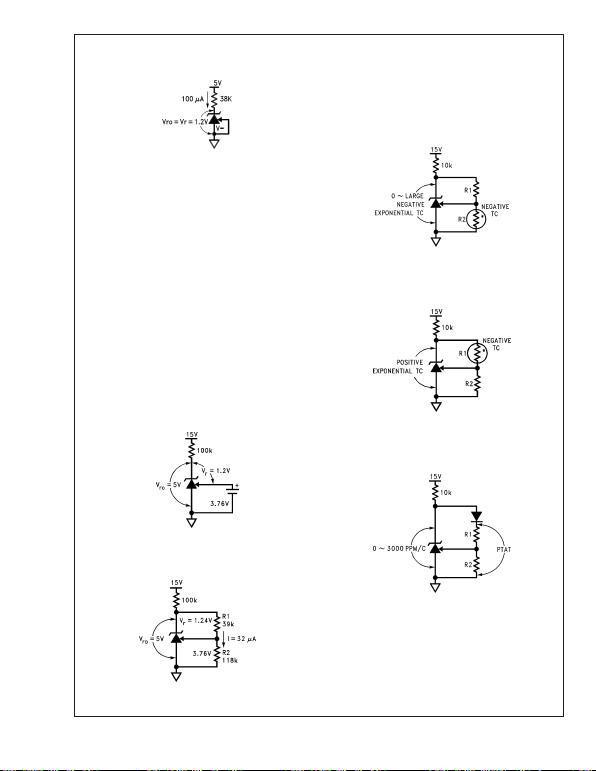Application Information (Continued)
Capacitors in parallel with the reference are allowed. See the
Reference AC Stability Range curve for capacitance
values from 20 µA to 3 mA any capacitor value is stable.
With the reference’s wide stability range with resistive and
capacitive loads, a wide range of RC filter values will perform
noise filtering.
The FEEDBACK pin allows the reference output voltage,
V
ro
, to vary from 1.24V to 6.3V. The reference attempts to
hold V
r
at 1.24V. If V
r
is above 1.24V, the reference will
conduct current from Cathode to Anode; FEEDBACK current
always remains low. If FEEDBACK is connected to Anode,
then V
ro
=V
r
= 1.24V. For higher voltages FEEDBACK is
held at a constant voltage above Anode say 3.76V for V
ro
= 5V. Connecting a resistor across the constant V
r
generates
a current I=R1/V
r
flowing from Cathode into FEEDBACK
node. A Thevenin equivalent 3.76V is generated from FEED-
BACK to Anode with R2=3.76/I. Keep I greater than one
thousand times larger than FEEDBACK bias current for
<0.1% error I32 µA for the military grade over the military
temperature range (I5.5 µA for a 1% untrimmed error for a
commercial part.)
00922118
R1 = Vr/I = 1.24/32µ = 39k
R2 = R1 {(Vro/Vr) 1} = 39k {(5/1.24) 1)} = 118k
Understanding that V
r
is fixed and that voltage sources,
resistors, and capacitors may be tied to the FEEDBACK pin,
a range of V
r
temperature coefficients may be synthesized.
Connecting a resistor across Cathode-to-FEEDBACK cre-
ates a 0 TC current source, but a range of TCs may be
synthesized.
00922116
FIGURE 3. 1.2V Reference
00922117
FIGURE 4. Thevenin Equivalent of
Reference with 5V Output
FIGURE 5. Resistors R1 and R2 Program
Reference Output Voltage to be 5V
00922119
FIGURE 6. Output Voltage has Negative Temperature
Coefficient (TC) if R2 has Negative TC
00922120
FIGURE 7. Output Voltage has Positive TC
if R1 has Negative TC
00922121
FIGURE 8. Diode in Series with R1 Causes
Voltage Across R1 and R2 to be Proportional
to Absolute Temperature (PTAT)
LM611
www.national.com 14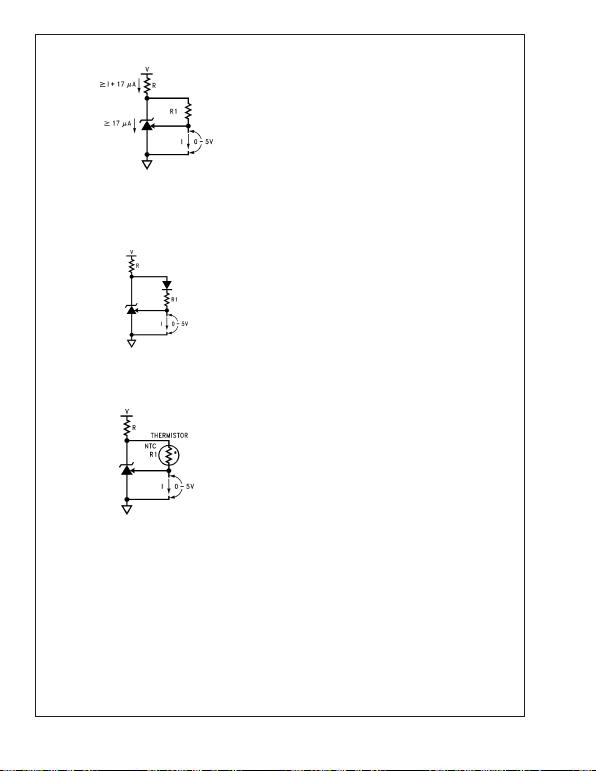Application Information (Continued)
00922122
I = Vr/R1 = 1.24/R1
Hysteresis
The reference voltage depends, slightly, on the thermal his-
tory of the die. Competitive micro-power products
vary always check the data sheet for any given device. Do
not assume that no specification means no hysteresis.
OPERATIONAL AMPLIFIER
The amp or the reference may be biased in any way with no
effect on the other, except when a substrate diode conducts
(see Guaranteed Electrical Characteristics Note 1). The amp
may have inputs outside the common-mode range, may be
operated as a comparator, or have all terminals floating with
no effect on the reference (tying inverting input to output and
non-inverting input to V
on unused amp is preferred).
Choosing operating points that cause oscillation, such as
driving too large a capacitive load, is best avoided.
Op Amp Output Stage
The op amp, like the LM124 series, has a flexible and
relatively wide-swing output stage. There are simple rules to
optimize output swing, reduce cross-over distortion, and op-
timize capacitive drive capability:
1. Output Swing: Unloaded, the 42 µA pull-down will bring
the output within 300 mV of V
over the military tempera-
ture range. If more than 42 µA is required, a resistor from
output to V
will help. Swing across any load may be
improved slightly if the load can be tied to V
+
, at the cost
of poorer sinking open-loop voltage gain.
2. Cross-over Distortion: The LM611 has lower cross-over
distortion (a 1 V
BE
BE
for the
LM124), and increased slew rate as shown in the char-
acteristic curves. A resistor pull-up or pull-down will force
class-A operation with only the PNP or NPN output
transistor conducting, eliminating cross-over distortion.
3. Capacitive Drive: Limited by the output pole caused by
the output resistance driving capacitive loads, a pull-
down resistor conducting 1 mA or more reduces the
output stage NPN r
e
until the output resistance is that of
the current limit 25. 200 pF may then be driven without
oscillation.
Op Amp Input Stage
The lateral PNP input transistors, unlike those of most op
amps, have BV
EBO
equal to the absolute maximum supply
voltage. Also, they have no diode clamps to the positive
supply nor across the inputs. These features make the in-
puts look like high impedances to input sources producing
large differential and common-mode voltages.
FIGURE 9. Current Source is Programmed by R1
00922123
FIGURE 10. Proportional-to-Absolute-
Temperature Current Source
00922124
FIGURE 11. Negative −TC Current Source
LM611
www.national.com15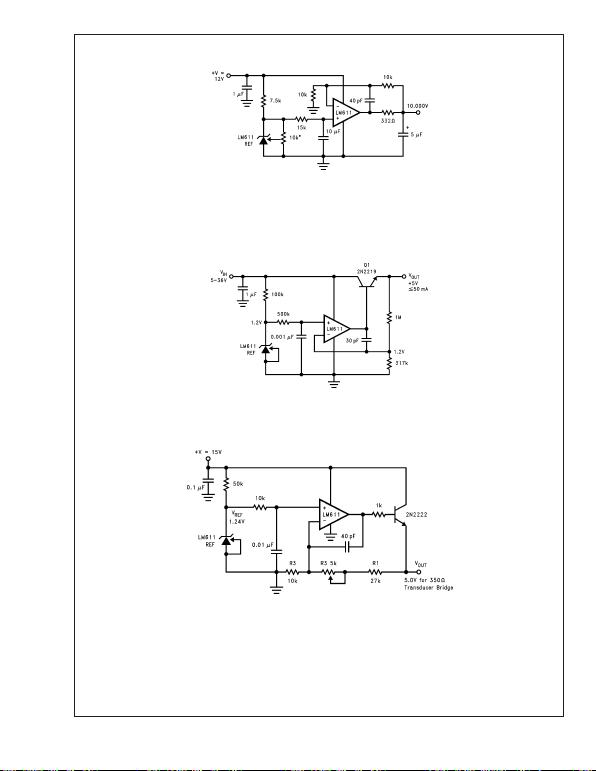Typical Applications
00922128
*10k must be low
t.c. trim pot.
FIGURE 12. Ultra Low Noise 10.00V Reference.
Total Output Noise is Typically 14 µV
RMS
.
Adjust the 10k pot for 10.000V.
00922130
FIGURE 13. Simple Low Quiescent Drain Voltage Regulator. Total Supply Current is approximately 320 µA when V
IN
=
5V, and output has no load.
00922129
VOUT = (R1/R2 + 1) VREF.
R1, R2 should be 1% metal film.
R3 should be low t.c. trim pot.
FIGURE 14. Slow Rise-Time Upon Power-Up,